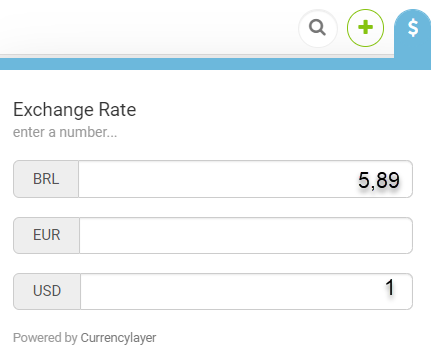Before proceeding with the instructions in this article, it is important to know that this is a very sensitive feature in relation to your pricing, so we recommend that you know the subjects below:

To correctly handle the default exchange rate on your Stays.net, it is important first to know a very basic concept: the exchange rate is changed every 24 hours, so you do not have full control over the result, but a tool to smooth out economic effects in your pricing, so we recommend using the feature only in last case, since the exchange rate of Stays.net is generated by the CurrencyLayer tool, one of the benchmarks in the market.

To calculate the percentage that will change the exchange rate of your Stays.net, follow the steps below:

Step
Summary
1What is the default currency of your listings?The first step in starting rate manipulation is to identify which currencies are your listings defaults.
Usually, it is a default currency that will serve as a basis for converting to other currencies.
As a practical example, we will assume our default currency is real (BRL) and we want to convert to US dollar (USD).
2Calculate the current exchange rate or a base rate you want to useThe math is simple: just pick 1 dollar (USD) and divide by the corresponding amount in your default currency.
As an example, we will use the system quotation and, from the currency calculator, we can check the quotation:The rate from the calculation would be 1/5,89, so it would be 0,1697792869269949.
3Do the same calculation, but for the rate you want to convertAssuming you want that on your Stays.net, the US dollar to be equivalent to 4 reais, just calculate 1/4 to generate the exchange rate.
In this case you would have 0,25 in the calculation.
4Divide the ideal rate by the current quotationIn our example, it would be 0,25 / 0,1697792869269949.
The result will be 1,4725.
5Subtract the rate by 1 and multiply by 100In our example, we got 1,4725 so when subtracting by 1, we have 0,4725.
By multiplying 0,4725 by 100, we get to 47,25!
6Round the result to insert into the systemStays.net will accept rate handling between 1 to 100%, integer amounts, so as we have 47,25% as a result, we will use 47% in the [BRLUSD] field in the [Multiplier for Exchange Rate].

Once you have obtained the rate, just enter it in the corresponding field in the [Multiplier for Exchange Rate] tool in your system settings.

The change will be applied in approximately 12 hours, for technical reasons.

If you do not know where to put the rate found in the calculation, take a look at the link below: